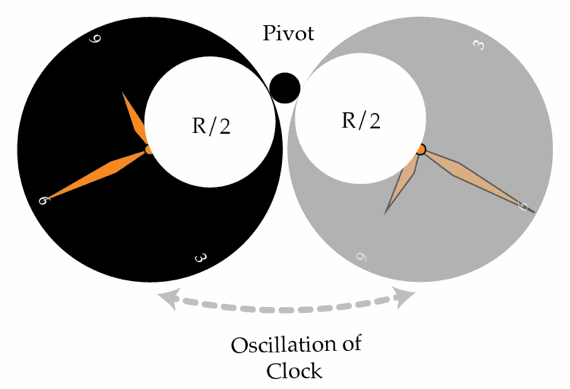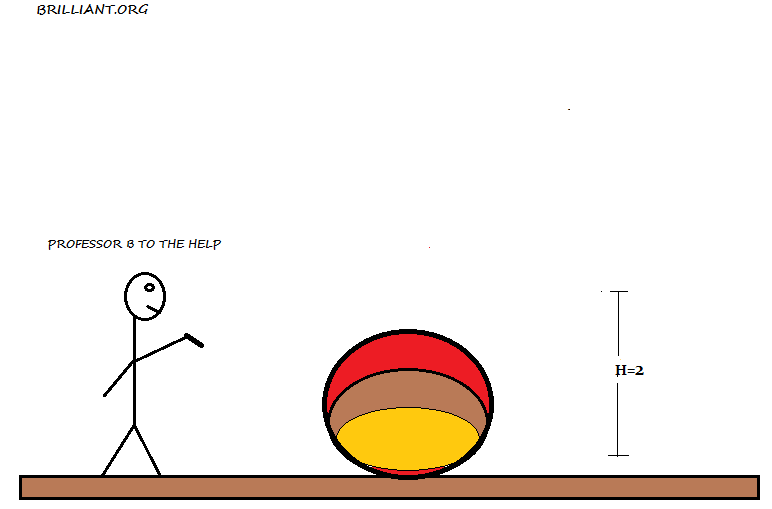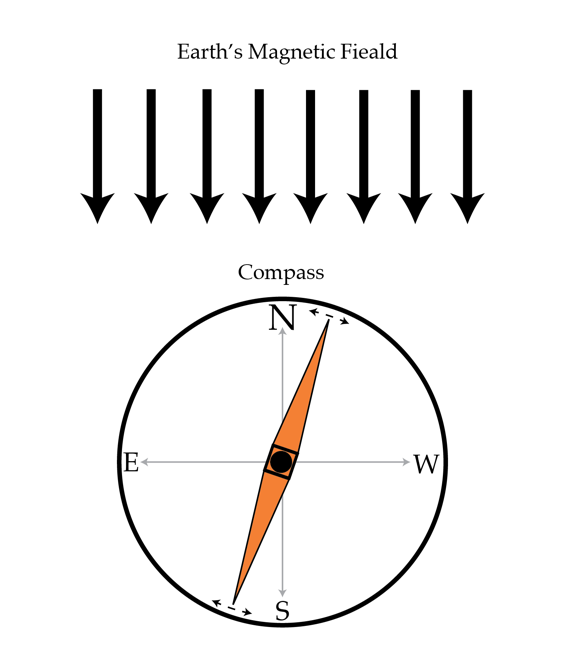Classical Mechanics

# Simple Harmonic Motion: Level 3-4 Challenges

An inventor designs a pendulum clock using a bob with mass 200 g at the end of a thin wire of length 23 cm. Instead of swinging back and forth, the bob is to move in a horizontal circle, making a fixed angle 27° with the vertical. This is called a conical pendulum because the suspending wire traces out a cone. Find the period $T$ of this pendulum.

Note: Use $\pi = 3.1416$ and $g = 9.81$ m/s$^2$.

A clock is made out of a disk of radius $R = 10~\mbox{cm}$ which is hung by a point on its edge and oscillates. All of a sudden, a circular part right next to the hanging point of radius $\frac{R}{2}$ falls off, but the clock continues oscillating. What is the absolute value of the difference in s between the periods of oscillation before and after the part fell off?Details and assumptions

• Gravitational acceleration is $g = 9.81~\mbox{m/s}^2$
• Amplitude of the vertical oscillations is small
• The axis of rotation of the disc is horizontal all the time
• The disc is homogeneousA ball of mass $1\textrm{ kg}$ is dropped from a height $H=2\textrm{ m}$. Estimate how long the ball is in contact with the ground (in seconds)?

Details and assumptions

• Simplify the question by modeling the ball as an ideal spring of spring constant $100 \textrm{ Nm}|$.
• If necessary, take the gravitational acceleration to be $9.8\textrm{ m/s}^2$.

A small magnetic needle in a compass performs small oscillations about an axis perpendicular to the Earth's magnetic induction field. On a different Earth location it is observed that the needle's oscillation period decreased by $\eta=1.5$ times, that is $\frac{T_{location 1}}{T_{location 2}}= 1.5.$ How much did the magnetic field of the earth change? In other words, determine $x=\frac{B_{location 2}}{B_{location 1}}.$ You may neglect the Earth's gravitational field in this problem.Not every oscillation in nature is a harmonic oscillation - in this problem, we will examine a non-harmonic oscillation. Suppose we had a rubber ball with a perfect coefficient of restitution so that, when dropped, it would always return to the same height. The period of the bouncing is the time $T$ between successive bounces and the amplitude $A$ of this non-linear oscillation is the maximum height of the ball above the floor. For a harmonic oscillator, the period and amplitude are independent, but not so here. Instead, there's a relation between them: $T$ proportional to $A^w$. Find $w$.

×